# GroupMe Analysis¶

## Introduction¶

This project uses GroupMe chat data (downloaded from https://web.groupme.com/profile/export) to extract insights from the electronic social interaction of our friend group (all names have been changed). This document features four parts. In part 1, the data is read in from a JSON file and extensively pre-processed for later analysis. Part 2 features advanced descriptive analysis, showcasing differences between chat members as well as heatmaps of interactions between members. It also features descriptive statistics on the chat in general, including plots of activity over time and optimal posting time. Part 3 features various Natural Language Processing (NLP) tasks aimed at extracting information from the messages themselves. Although some of this code has been adapted specifically for this chat, I am working on packaging all code into functions and creating a website that integrates with GroupMe's API so that others may access analytics on their chats.

## 1. Setup¶

### 1.1 Imports and Read in Data¶

This script relies on a large amount of excellent python libraries, but most significant are sklearn (for machine learning), nltk (for natural language processing), matplotlib (for data visualization), numpy (for numerical computation and data structures), and pandas (for data structures and many helpful shortcut functions). The reader is encouraged to consult the thorough online documentation of these libraries for areas of the following code that may be unclear.

In :
from sklearn.naive_bayes import MultinomialNB, BernoulliNB
from sklearn.linear_model import LogisticRegression, SGDClassifier
from sklearn.svm import SVC, LinearSVC, NuSVC
from sklearn.feature_extraction.text import CountVectorizer,TfidfVectorizer
from nltk.corpus import stopwords
from nltk.tokenize import word_tokenize
from nltk.classify import ClassifierI
from nltk.classify.scikitlearn import SklearnClassifier
import json
import matplotlib.pyplot as plt
import os
import numpy as np
import pandas as pd
from datetime import datetime as dt
from dateutil.parser import parse
import matplotlib.dates as mdates
import random
import pickle
from statistics import mode
import re
import matplotlib.style as style
import warnings
from sklearn.ensemble import RandomForestRegressor
from rake_nltk import Metric,Rake
import time
import seaborn as sns
from wordcloud import WordCloud,STOPWORDS
import scipy.stats as stats
from textblob import TextBlob
import operator

warnings.filterwarnings('ignore')

In :
os.chdir('C:\\Users\\Ben Gochanour\Documents\\Past Semesters\Fall 2019\Heckin Nerds Project')


### 1.2 Data Cleaning and Calculated Columns¶

Although the data exists natively in a pretty useful format, here we add additional calculated columns and remove extraneous information.

In :
values=[] # Get attachment indicator column
for i in my_data["attachments"]:
if len(i)>0:
value=1
else:
value=0
values.append(value)

my_data['attachment']=values

In :
my_data['nickname']=my_data['name'] # copy name col and rename 'nickname'

In :
ids=my_data['sender_id'].unique()
my_data = my_data[['created_at','favorited_by','nickname','name','sender_id','text','attachment']]


The data includes both names and sender ids to identify users. However, there is not a one-to-one correspondence between name and user because users accumulate many names as they change their nickname in the group. Therefore, we will map the sender_id (which is unique for each person) to useful names. In general, this code will just pull the first nickname for each user as their final name mapping, although for this group I have provided manual specification of preferred names.

In :
names=[] # Create name dictionary, in general manual specification wouldn't be done but allows for better customization here
for id in ids:
df=my_data[my_data['sender_id']==id]
name=df['name']
if len(name)>0:
name=name.iloc
else:
name="Blank"
names.append(name)

dictionary = dict(zip(ids,names))

dictionary['41020482']='John'
dictionary['47893872']='Tyler'
dictionary['50618759']='Bob'
dictionary['30132924']='Steve'
dictionary['28346711']='Xavier'
dictionary['14475811']='Michael'
dictionary['39762430']='Thomas'
dictionary['48038657']='Sean'
dictionary['28278581']='Joe'
dictionary['33483318']='Christian'
dictionary['38876568']='Aaron'
dictionary['calendar']='Calendar'
dictionary['17296420']='Victor'
dictionary['49894572']='Jalen'
dictionary['38876567']='Jason'

my_data["name"] = my_data["sender_id"].map(dictionary)

In :
dictionary

Out:
{'system': 'GroupMe',
'33483318': 'Christian',
'47893872': 'Tyler',
'41020482': 'John',
'38876568': 'Aaron',
'38876567': 'Jason',
'50618759': 'Bob',
'30132924': 'Steve',
'28346711': 'Xavier',
'14475811': 'Michael',
'39762430': 'Thomas',
'48038657': 'Sean',
'28278581': 'Joe',
'calendar': 'Calendar',
'17296420': 'Victor',
'49894572': 'Jalen'}
In :
my_data.columns=['send_time','liked_by','nickname','name','sender_id','message','attachment']

lengths=[]
for i in my_data["liked_by"]:
length=len(i)
lengths.append(length)

my_data['n_likes']=lengths

In :
def datetime_from_utc_to_local(utc_datetime): # Times are in UTC, convert to whatever user's local time zone is
now_timestamp = time.time()
offset = dt.fromtimestamp(now_timestamp) - dt.utcfromtimestamp(now_timestamp)
return utc_datetime + offset

my_data['send_time']=datetime_from_utc_to_local(my_data['send_time'])

In :
# Remove these members
my_data=my_data[my_data['name']!='Calendar']
my_data=my_data[my_data['name']!='GroupMe']
my_data=my_data[my_data['name']!='Victor']
my_data=my_data[my_data['name']!='Jalen']

In :
ids_new=my_data.sender_id.unique()

In :
nicknames=[] #create list of nicknames with @ for later use
for name in my_data['name'].unique():
if name not in ["Jalen","Victor"]:
subset=my_data[my_data.name==name]
my_nicknames=list(subset.nickname.unique())
my_nicknames=["@"+s for s in my_nicknames]
nicknames.append(my_nicknames)


In :
lengths=[len(i) for i in nicknames] #put this info into a data frame
nicknames_df=pd.DataFrame({'length':lengths,'name':my_data["name"].unique()})

In :
my_data=my_data.reset_index(drop=True) # Did lots of subsetting so call this to reset everything


## 2. Descriptive Analysis¶

### 2.1 Individual Analysis¶

At this point, we might be interesting in learning about the members of our chat. Who is the most popular? The most social? Etc. To do this we will rely heavily on value_counts and other data manipulation methods available in pandas.

MOST POPULAR

In :
names_group=my_data.groupby('name')
data=names_group['n_likes'].mean()
data=pd.DataFrame(data)
data.columns=['avg likes']

fig=data.sort_values(by='avg likes',ascending=False).plot(kind='bar',
title='Avg. Likes Recieved by Member',legend=False)

fig.set_xlabel("Member")
fig.set_ylabel("Average Like Count")

Out:
Text(0, 0.5, 'Average Like Count')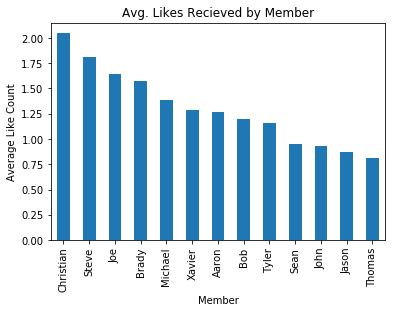MOST TALKATIVE

In :
fig=my_data['name'].value_counts().plot(kind="bar",title="Messages Sent by Member")
fig.set_ylabel("Messages Sent")
fig.set_xlabel("Member")
total=len(my_data)
my_str='Total='+str(total)
fig.annotate(my_str,xy=(13,1540))

Out:
Text(13, 1540, 'Total=14360')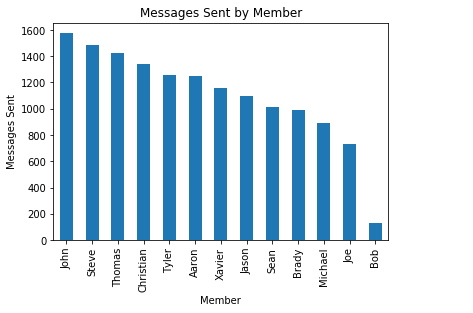MOST VERBOSE

In :
my_data['message_len']=my_data.message.str.split().str.len()
fig=my_data.groupby('name').message_len.mean().sort_values(ascending=False).plot(kind="bar",title="Average Message Length")
fig.set_xlabel("Member")
fig.set_ylabel("Number of Words")

Out:
Text(0, 0.5, 'Number of Words')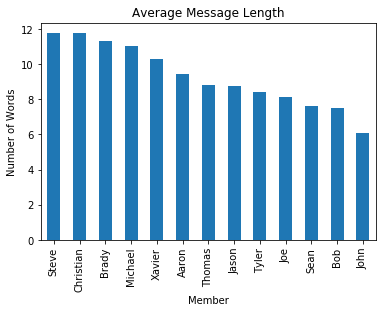MOST SUPPORTIVE

To decide who is the most supportive, we'd like to look at the rate at which users like other users' messages. However, at this point we are faced with a problem: users have been in the chat for a different amount of time, and thus have seen different amount of methods. Therefore, we will factor this into our computations by computing "messages seen" counts for each user.

In :
new_list=my_data[["liked_by"]]
new_list=[i for i in new_list['liked_by']]
flat_list=[item for sublist in new_list for item in sublist]
df=pd.DataFrame(flat_list,columns=["id"])
next_step=df.id.value_counts()
df=pd.DataFrame(next_step)
df['name']=[i for i in df.index]

In :
my_names=sorted(my_data['name'].unique())
seen=[]
for name in my_names:
subset=my_data[my_data.name==name]
value=(max(subset.index))
seen.append(value)

In :
df=df.reset_index(drop=True)

In :
df["name"] = df["name"].map(dictionary)
df.columns=['n',"name"]
df=df[df.name!="Victor"]
df=df[df.name!="Jalen"]
df.sort_values(by="name",inplace=True)
df=df.reset_index(drop=True)
df['avg']=[df['n'][i]/seen[i] for i in range(len(seen))]
fig=df.sort_values(by='avg',ascending=False).plot(x="name",y="avg",kind="bar",legend=False,title="Like Rate by Member")
fig.set_xlabel("Member")
fig.set_ylabel("Like Rate")

Out:
Text(0, 0.5, 'Like Rate')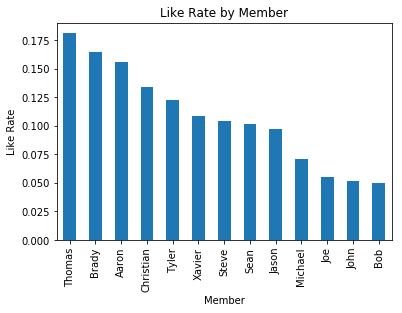MOST SOCIAL

In :
ats=my_data[my_data.message.str.contains("@")==True]
#1421 total ats
fig=ats.groupby("name").send_time.count().sort_values(ascending=False).plot(kind="bar",legend=False,
title="Frequency of Mentioning Other Members")
fig.set_xlabel("Member")
fig.set_ylabel("Number of Mentions of Others")

Out:
Text(0, 0.5, 'Number of Mentions of Others')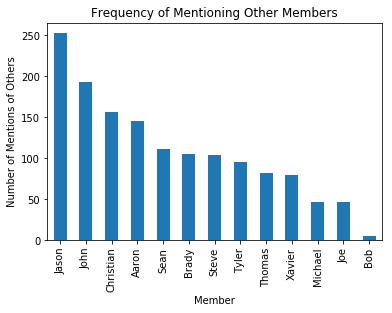MOST INDECISIVE

In :
fig=nicknames_df.set_index("name").sort_values(by="length",ascending=False).plot(kind="bar",title="Most Nicknames Used",
legend=False)
fig.set_xlabel("Member")
fig.set_ylabel("Number of Nicknames")

Out:
Text(0, 0.5, 'Number of Nicknames')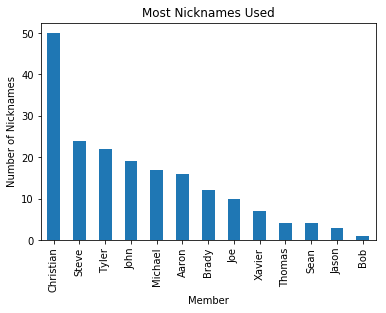### 2.2 Paired Analysis¶

Thus far we have looked at individual users, but we can also look at combinations between pairs of users. First, we may be interested in examining who likes whose messages. To do this, we will perform some computations and plot a heatmap to see the like rates among all pairwise combinations of users. Note that this calculation also accounts for the fact that some users have seen more messages than others. This analysis is (relatively) computationally expensive, although with only partially optimized code a chat with 15,000 messages and 13 members runs in about .18 seconds.

In :
liking=pd.DataFrame(columns=my_names,index=my_names)
liking_freq=pd.DataFrame(columns=my_names,index=my_names)

In :
my_data=my_data.reset_index(drop=True)

In :
for name in my_data["name"].unique():
subset=my_data[my_data["name"]==name]
len_subset=len(subset)
new_list=subset[["liked_by"]]
new_list=[i for i in new_list['liked_by']]
flat_list=[item for sublist in new_list for item in sublist]
df=pd.DataFrame(flat_list,columns=["n"])
next_step=pd.DataFrame(df.n.value_counts())
for id in ids_new:
if id not in next_step.index:
next_step=next_step.append(pd.Series(name=id))
next_step['n'][id]=0
next_step['id']=next_step.index
next_step['name']=next_step.id.map(dictionary)
next_step.sort_values(by="name",inplace=True)
next_step['percentage']=round(next_step['n']/len_subset*100,1)
if '17296420' in next_step.index: #Slight time boost over try_except here
next_step.drop('17296420',inplace=True)
if '49894572' in next_step.index:
next_step.drop('49894572',inplace=True)

next_step=next_step.reset_index(drop=True)

next_step['factor']=[len(my_data)/seen[i] for i in range(len(next_step))]
next_step['new_percentage']=[next_step['percentage'][i]*next_step['factor'][i] for i in range(len(next_step))]

liking[name]=next_step.new_percentage.values
liking_freq[name]=next_step.n.values

In :
liking=liking.astype('float')
fig=sns.heatmap(liking,annot=True)
fig.set_xlabel("Message Posted by")
fig.set_ylabel("Like Rate by Member")
fig.set_title("Like Rate Heatmap",fontweight="bold")

Out:
Text(0.5, 1.0, 'Like Rate Heatmap')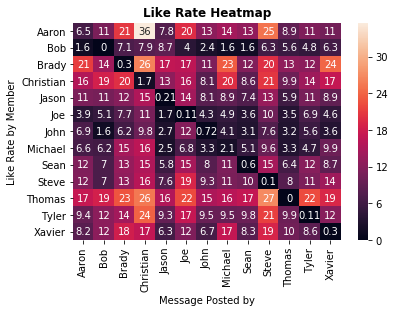Here, each user can see both their biggest fans as well as the people whose messages they tend to like at the highest rates. We can also see a person's like rate of their own messages, which in general is quite low, although there are some exceptions. Next, we can look at mentions (@s) as a similar method for better understanding the interaction between users.

In :
ating_freq=pd.DataFrame(columns=my_names,index=my_names)

In :
for idx,name in enumerate(my_data["name"].unique()):
subset=my_data[my_data.name==name]
len_subset=len(subset)
search_list= nicknames[idx]
my_data['c'] = my_data.message.str.extract('({0})'.format('|'.join(search_list)), flags=re.IGNORECASE)
df = my_data[~pd.isna(my_data.c)]
next_step=df.name.value_counts().to_frame("n")

for person in my_names:
if person not in next_step.index:
next_step=next_step.append(pd.Series(name=person))
next_step['n'][person]=0

next_step['name']=next_step.index
next_step.sort_values(by="name",inplace=True)

next_step['percentage']=round(next_step['n']/len_subset*100,1)

if 'Jonah' in next_step.index: #Slight time boost over try_except here
next_step.drop('Jalen',inplace=True)
if 'Erik' in next_step.index:
next_step.drop('Jason',inplace=True)

next_step=next_step.reset_index(drop=True)

next_step['factor']=[len(my_data)/seen[i] for i in range(len(next_step))]
next_step['new_percentage']=[next_step['percentage'][i]*next_step['factor'][i] for i in range(len(next_step))]

ating_freq[name]=next_step.n.values

In :
ating_freq=ating_freq.astype('float')
fig=sns.heatmap(ating_freq,annot=True)
fig.set_xlabel("Person Being Mentioned")
fig.set_ylabel("Mentions of Others")
fig.set_title("Total Mentions Heatmap",fontweight="bold")

Out:
Text(0.5, 1.0, 'Total Mentions Heatmap')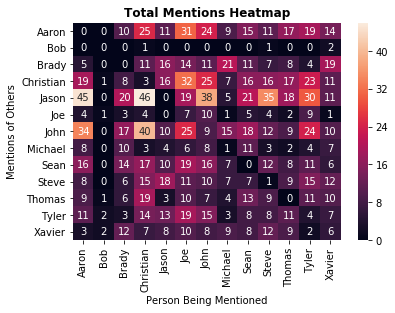### 2.3 Chatwide Statistics¶

The previous analysis has covered individuals and pairs of individuals, but we may be interested in better understanding the chat as a whole. First, we will approximate the joining date of each member using the data of their first message sent.

In :
# Used first message sent date as proxy for joined data
dates=[]
for i in seen:
date=my_data.send_time[i]
dates.append(date)

In :
pd.DataFrame({'name':my_names,'joined':dates}).sort_values(by="joined").reset_index(drop=True)

Out:
name joined
0 Christian 2017-10-30 18:15:38
1 Sean 2017-11-01 23:51:37
2 Michael 2017-11-01 23:53:51
3 Steve 2017-11-02 00:08:17
4 Xavier 2017-12-15 10:17:31
6 John 2018-02-22 18:40:17
7 Jason 2018-04-13 21:01:54
8 Tyler 2018-04-13 21:15:44
9 Joe 2018-04-27 15:35:00
10 Aaron 2018-04-29 14:53:51
11 Thomas 2018-05-11 06:40:58
12 Bob 2019-08-16 21:43:37

Here, we see clusters of numerous adds, especially in April-May, 2018.

To gauge overall engagement with the chat, we can plot the frequency of various like counts.

In :
fig=my_data['n_likes'].value_counts().plot(kind="bar",title="Likes Frequency Plot")
fig.set_ylabel("Frequency")
fig.set_xlabel("Number of Likes")

Out:
Text(0.5, 0, 'Number of Likes')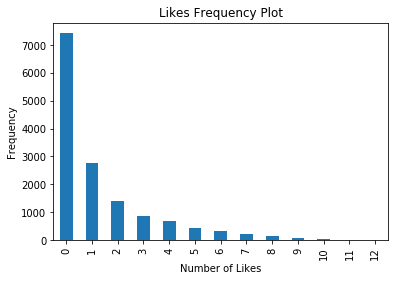We see a highly skewed right distribution, as it is very common for messages to obtain a small number of likes. Next we would like to look at the 10 most-liked messages of all time. Note that 'None' indicates that it was a message sent with an attachment only.

How have avg. likes changed over time?

In :
time_indexed=my_data.set_index('send_time')
fig=time_indexed.resample('M').n_likes.mean().plot(kind="line",legend=False ,title="Average Likes by Message Posting Month")
fig.set_xlabel('Month')
fig.set_ylabel('Average Likes')
fig

Out:
<matplotlib.axes._subplots.AxesSubplot at 0x1ea10603eb8>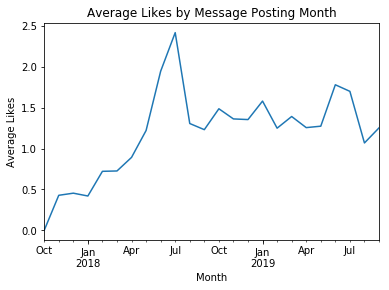How has number of messages sent per month changed over time?

In :
# How has activity changed over time? Notice dips during breaks but overall increase
fig=time_indexed.resample('M').message.count().plot(legend=False,kind='line',title="Number of Messages Sent Per Month")
fig.set_xlabel('Month')
fig.set_ylabel('Number of Messages')

Out:
Text(0, 0.5, 'Number of Messages')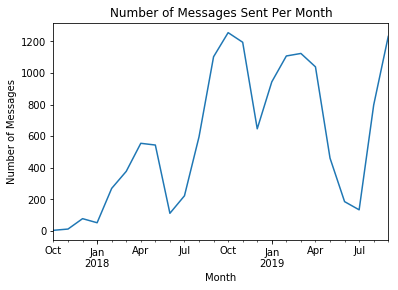In :
# When is the best posting time?
# Number of messages sent/hr over the past few days
fig=time_indexed.groupby(time_indexed.index.hour).n_likes.mean().plot(title='Optimal Posting Time for Likes')
fig.set_xlabel("24-hr time")
fig.set_ylabel('Average likes')
fig=fig.set_xticks(range(0,23,2))
fig

Out:
[<matplotlib.axis.XTick at 0x1ea105582e8>,
<matplotlib.axis.XTick at 0x1ea10558828>,
<matplotlib.axis.XTick at 0x1ea10545a58>,
<matplotlib.axis.XTick at 0x1ea10540e48>,
<matplotlib.axis.XTick at 0x1ea105403c8>,
<matplotlib.axis.XTick at 0x1ea10540dd8>,
<matplotlib.axis.XTick at 0x1ea10558fd0>,
<matplotlib.axis.XTick at 0x1ea10534f60>,
<matplotlib.axis.XTick at 0x1ea10534780>,
<matplotlib.axis.XTick at 0x1ea10534198>,
<matplotlib.axis.XTick at 0x1ea1052ff60>,
<matplotlib.axis.XTick at 0x1ea1052f4e0>]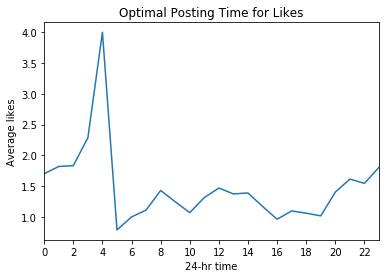When is the chat most active overall?

In :
# Chat is least active in the morning and peaks at night, need to change this to average by getting n days
fig=time_indexed.groupby(time_indexed.index.hour).message.count().plot(title='Activity of Chat')
fig.set_xlabel("24-hr time")
fig.set_ylabel('Messages Sent')

Out:
Text(0, 0.5, 'Messages Sent')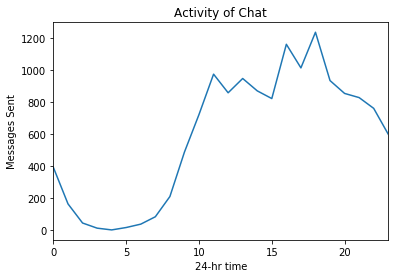How does the activity of the chat relate to like rate?

In :
# This is a good way to combine above too charts, thing is I need to avg this messages sent
fig, ax1 = plt.subplots()
data1=time_indexed.groupby(time_indexed.index.hour).n_likes.mean()
ax1.plot(data1,color="orange")
ax1.set_xlabel("24-hr time")
ax1.set_ylabel("Avg Likes")

ax2 = ax1.twinx()  # instantiate a second axes that shares the same x-axis
data2=time_indexed.groupby(time_indexed.index.hour).message.count()
ax2.plot(data2,color="blue")
ax2.set_ylabel('Number of Messages Sent')

fig.tight_layout()
plt.show()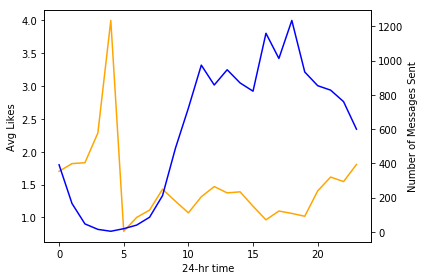The two variables appear to be inversely related.

## 3. Textual Analysis¶

### 3.1 Basic Textual Analysis¶

Before processing our textual data, we can look at the most frequent (exact) messages sent.

In :
fig=my_data['message'].value_counts().head(15).plot(kind='bar',title='Most Frequent (Exact) Messages Sent')
fig.set_ylabel('Count')
fig.set_xlabel('Message')

Out:
Text(0.5, 0, 'Message')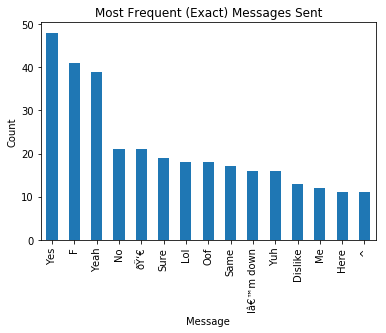However, standardizing our data by removing uppercase characters and correcting issues we notice with character encodings will allow for better analysis later on. To this point, I don't have an effective way to deal with emojis, although I am working on this.

In :
processed_features = []

features=my_data['message']
features=features.reset_index(drop=True)

# Processing in a custom way for this data, may have to change later
for sentence in range(0, len(features)):

# Remove all the special characters

#processed_feature = re.sub(r'\W', ' ', str(features[sentence]))
processed_feature=str(features[sentence])
#Substituting multiple spaces with single space
processed_feature = re.sub(r'\s+', ' ', processed_feature, flags=re.I)
# Converting to Lowercase
processed_feature = processed_feature.lower()

processed_feature=processed_feature.replace("â ","'")
processed_feature=processed_feature.replace("â€™","'")

processed_features.append(processed_feature)


As a quick visualization of our results post-processing, we can create a quick wordcloud to show the most common words used in chat messages.

In :
text=processed_features
result= ''
for element in text:
result += " "+str(element)
result

# Create the wordcloud object
wordcloud = WordCloud(width=480, height=480, margin=0,stopwords=STOPWORDS).generate(result)
plt.imshow(wordcloud, interpolation = 'bilinear')
plt.axis('off')
plt.show()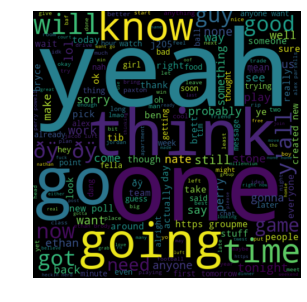The Rapid Automatic Keyword Extraction (RAKE) Algorithm seeks to determine key phrases in a body of text by analyzing word appearance frequency and common word co-occourances (Rose et. al, 2010). This project will use the rake-nltk implementation (v. 1.0.4).

In :
# Implement RAKE Algorithm, not getting good results yet but keep for now

rake_object = Rake(language="English", ranking_metric=Metric.WORD_FREQUENCY, min_length=1, max_length=10) #use English stopwords from NLTK and all punctuation characters

def RAKE_keyword_extract(dataset,rake_object): #implement RAKE keyword extraction algorithm
for rcol in dataset.columns:
rake_var = dataset[rcol].replace(to_replace=['(?i)None','(?i)NA', '(?i)N/A','(?)No'], value=np.nan).dropna() #replace 'None' and 'N/A' strings in free-text fields with missing value (None)
rake_var.replace(to_replace=['T/O','t/o'], value='takeoff', regex=True, inplace=True)
if(rake_var.shape==0):
continue
else:
var_temp = np.array(rake_var) #convert free-text fields to arrays
var_keyword_list = pd.DataFrame(index=None) #get keywords from free-text entries

for indx in range(0,rake_var.shape):
rake_object.extract_keywords_from_text(str(var_temp[indx])) #extract keywords/key phrases from free text field using RAKE
rkobj=pd.DataFrame(rake_object.get_ranked_phrases_with_scores()) #rank keywords by degree-to-frequency ratio
if rkobj.empty:
continue
else:
rkobj.columns = ['Word_frequency','Key_phrase']
rkobj['Record_number'] = rake_var.index[indx]
var_keyword_list = var_keyword_list.append(rkobj)
var_keyword_list = var_keyword_list[var_keyword_list.columns[[2,1,0]]]
var_keyword_list.to_csv("RAKE_Keywords_" + str(rcol) + ".csv",sep=',')

str1 = ''.join(processed_features)
rake_object.extract_keywords_from_text(str1) #extract keywords/key phrases from free text field using RAKE
rkobj=pd.DataFrame(rake_object.get_ranked_phrases_with_scores())


RAKE is selecting emoji-laden messages as most significant. Because these have not been read into Python perfectly, we can't do much analysis here. However, I will keep this code in for use with other chats.

We can also adopt a more specific approach by getting the most frequent "n-grams", that is, strings of length n. Below, we will take a look at n=1 and n=3. It is important to note that this implementation removes stopwords, which include common words like "a", "an", and "the". This step serves to ensure that we focus our interpretation on the most important information.

In :
pattern="(?u)\\b[\\w']+\\b"
def get_top_n_mgram(corpus, n, m):
vec = CountVectorizer(stop_words="english",ngram_range=(m,m),token_pattern=pattern).fit(corpus)
bag_of_words = vec.transform(corpus)
sum_words = bag_of_words.sum(axis=0)
words_freq = [(word, sum_words[0, idx]) for word, idx in vec.vocabulary_.items()]
words_freq =sorted(words_freq, key = lambda x: x, reverse=True)
return words_freq[:n]

In :
common_words = get_top_n_mgram(processed_features, 20,1)
df5 = pd.DataFrame(common_words, columns = ['Text' , 'Count'])
fig=df5.groupby('Text').sum()['Count'].sort_values(ascending=False).plot(
kind='bar',title='Top 20 Unigrams after removing stop words')
fig.set_xlabel("Unigram")
fig.set_ylabel("Frequency")

Out:
Text(0, 0.5, 'Frequency')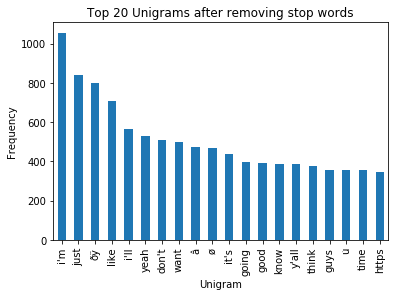In :
common_words = get_top_n_mgram(processed_features, 20,3)
df5 = pd.DataFrame(common_words, columns = ['Text' , 'Count'])
fig=df5.groupby('Text').sum()['Count'].sort_values(ascending=False).plot(
kind='bar',title='Top 20 Trigrams in Messages after removing stop words')
fig.set_xlabel("Trigram")
fig.set_ylabel("Frequency")

Out:
Text(0, 0.5, 'Frequency')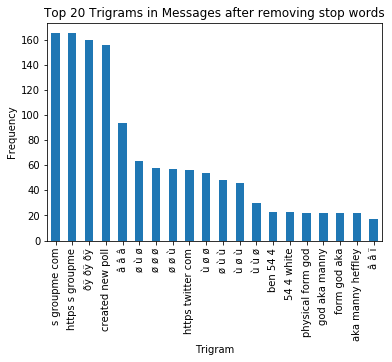We can also examine other parts of the text, including the most common parts of speech used. Unsurprisingly, nouns are by far the most common. So far this analysis is lacking a point of comparison, although after more chats are run through this tool their exists the possibility of establishing a meaningful standard for GroupMe data.

In :
blob = TextBlob(str(processed_features))
pos_df = pd.DataFrame(blob.tags, columns = ['word' , 'pos'])
pos_df = pos_df.pos.value_counts()[:20].to_frame(name="n")

In :
pos_dict={'NN': 'noun',
'IN': 'prep./subord. conj.',
'VB': 'verb-base form',
'DT': 'determiner',
'PRP': 'pers. pronoun',
'VBP': 'verb-sing. pres.',
'NNS': 'plural noun',
'VBZ': 'verb-3rd pers. sing.',
'TO':"to",
'VBD': 'verb-past tense',
'MD': 'modal',
'CD': 'cardinal digit',
'NNP': 'prop. noun-singular'}

pos_df.index=pos_df.index.map(pos_dict)

In :
fig=pos_df.head(15).plot(kind="bar",title="Frequency of Different Parts of Speech in Chat",legend=False)
fig.set_xlabel("Part of Speech")
fig.set_ylabel("Frequency")

Out:
Text(0, 0.5, 'Frequency')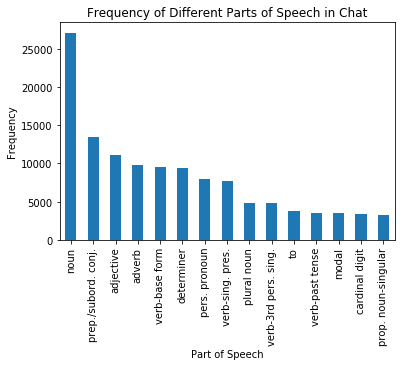Thus far, we have looked at all approximately 15,000 messages together. However, as in part one of this analysis, we may be interested in individual-level data. TFIDF (term frequency-inverse document frequency) is a numerical statistic frequently used to analyze how important a word is to a specific document within a larger collection of documents (sometimes called a corpus). For each document, the TDIF value of a word increases proportionally to its occurance frequency in that document, although it is offset by how many times the word appears in the full corpus. In this way, common words are less likely to be selected by simple virtue of being common. Hence, TFIDF is an extremely useful statistic that is widely used; in fact, 83% of text-based recommender systems in digital libraries use TFIDF (Breitinger, 2015).

Here a "document" is all of one user's messages and the corpus is the collection of all such documents. The TFIDF vectorizer used here is the implementation available in sklearn. Please note that the auxillary code for this task is adapted from github user jldbc and is available at https://github.com/jldbc/groupme-analytics/blob/master/Groupme_Analytics.ipynb.

In :
my_data=my_data.reset_index(drop=True)
my_data=my_data.sort_values(by="send_time",ascending=False)
my_data['message_cleaned']=processed_features

In :
documents = []
for i in my_data["name"].unique():
document = ""
msgs = my_data[my_data['name']==str(i)]['message_cleaned']
for i in msgs:
document += (str(i) + " ")
documents.append(document)

#create the vectorizer
tfidf = TfidfVectorizer(max_df=0.9,
ngram_range=(1, 1),
stop_words='english',
strip_accents=None, analyzer = 'word')

#run the multiplicaiton
tfidf_matrix =  tfidf.fit_transform(documents)
feature_names = tfidf.get_feature_names()

In :
my_words=[]
scores=[]
names=[]
indices = np.argsort(tfidf.idf_)#[::1]
tfidf_matrix_2 = tfidf_matrix.todense()
top_n = 100
top_features = [feature_names[i] for i in indices[:top_n]]
i = 0
j = 0
dicts = []
for person in my_data["name"].unique():
persondict = {}
for word in feature_names:
if tfidf_matrix[i,j] != 0:
persondict[word] = tfidf_matrix[i,j]
j += 1
j = 0
i += 1
#print('person done')
dicts.append(persondict)
#sorted(tfidf_matrix_2.tolist())[::-1]

j = 0
words_dict = {}
for i in dicts:
sorted_vals = sorted(i.items(), key=operator.itemgetter(1), reverse=True)
words_dict[str(my_data["name"].unique()[j])] = sorted_vals[0:15]
j += 1

for entry in words_dict:
for w in words_dict[entry]:
my_words.append(w)
scores.append(w)
names.append(entry)

In :
my_df=pd.DataFrame({'name':names,'word':my_words,'score':scores})

In :
subset=my_df[my_df.name=="Xavier"]
fig=subset.plot(kind="bar",legend=False,title=str(name)+" Highest Tf-idf scores")
fig.set_xlabel("Word")
fig.set_ylabel("Score")
fig.set_xticklabels(subset.word)

Out:
[Text(0, 0, 'lol'),
Text(0, 0, 'brett'),
Text(0, 0, 'fellas'),
Text(0, 0, 'tim'),
Text(0, 0, 'hello'),
Text(0, 0, '19'),
Text(0, 0, 'status'),
Text(0, 0, 'nathan'),
Text(0, 0, 'tickets'),
Text(0, 0, 'lmao'),
Text(0, 0, 'thinking'),
Text(0, 0, 'lmaoo'),
Text(0, 0, 'gotcha'),
Text(0, 0, 'appreciate')]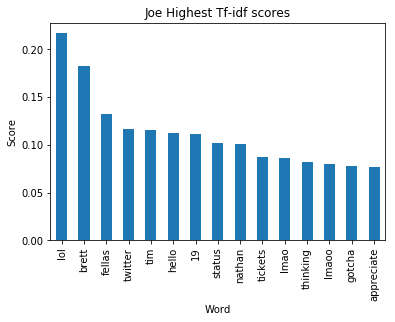One member is shown above as an example, could also run a for loop to show all members. Knowing this member, these results make sense.

### 3.2 Sentiment Analysis¶

Sentiment analysis is an NLP technique that attempts to extract information on the sentiment (emotional tone) of a piece of text, where -1 represents fully negative, 1 represents fully positive, and 0 is neutral.

In :
sid = SentimentIntensityAnalyzer()

sentiment=[]
for i in processed_features:
my_score=sid.polarity_scores(i)['compound']
sentiment.append(my_score)

my_data['sentiment']=sentiment

In :
# Checking some of Vader's properties, format into Latex table eventually

#Has texting acronyms and can recognize regular typed emojis
sid.polarity_scores('Lmao jk')['compound']
sid.polarity_scores('smh')['compound']
sid.polarity_scores(':)')['compound']
sid.polarity_scores(':/')['compound']

# Punctuation counts: exclamation yields higher score
sid.polarity_scores('Thanks')['compound']
sid.polarity_scores('Thanks!')['compound']

Out:
0.4926
In :
fig=my_data.sentiment.plot(kind="hist",title="Histogram of Sentiment Scores")
fig.set_xlabel("Sentiment Score")

Out:
Text(0.5, 0, 'Sentiment Score')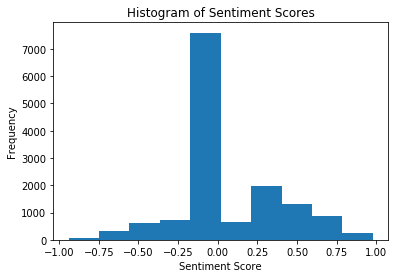MOST POSITIVE

In :
fig=my_data.groupby('name').sentiment.mean().sort_values(ascending=False).plot(kind="bar",title="Average Sentiment Score by Member")
fig.set_xlabel("Member")
fig.set_ylabel('Avg. Sentiment Score')

Out:
Text(0, 0.5, 'Avg. Sentiment Score')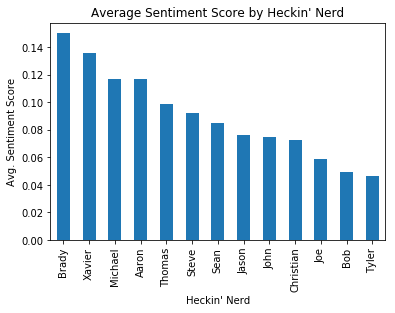What day of the week are people most positive?

In :
# This is for all
week_dictionary={0:"Mon",1:"Tues",2:"Wed",3:"Thurs",4:"Fri",5:"Sat",6:"Sun"}
my_data['weekday'] = my_data['send_time'].dt.dayofweek
my_data['weekdaynew']=my_data['weekday'].map(week_dictionary)
testing=my_data.sort_values(by='weekday')
fig=testing.groupby('weekdaynew',sort=False).sentiment.mean().plot(kind="bar",title="Avg. Sentiment Score by Day of Week")#Monday=0, Sunday=6
fig.set_xlabel('Day of Week')
fig.set_ylabel('Sentiment Score')

Out:
Text(0, 0.5, 'Sentiment Score')